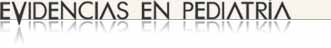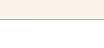# Evaluation of the accuracy of diagnostic tests (1). Discrete variables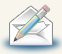Regularly recieve most recent articles by e-mail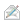Subscribe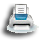Print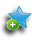Add to library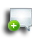Discuss this article

### MBE | Bases of evidence based medicine

Authors: Ochoa Sangrador C1, Molina Arias M2.
1Servicio de Pediatría. Hospital Virgen de la Concha. Zamora. España.
2Servicio de Gastroenterología. Hospital Infantil Universitario La Paz. Madrid. España.
Correspondence: Carlos Ochoa Sangrador. Email: cochoas2@gmail.com
Reception date: 20/05/2017
Acceptance date: 24/05/2017
Publication date: 31/05/2017

Ochoa Sangrador C, Molina Arias M. Evaluación de la precisión de las pruebas diagnósticas (1). Variables discretas. Evid Pediatr. 2017;13:28.

### MBE | Bases of evidence based medicine

Authors: Ochoa Sangrador C1, Molina Arias M2.
1Servicio de Pediatría. Hospital Virgen de la Concha. Zamora. España.
2Servicio de Gastroenterología. Hospital Infantil Universitario La Paz. Madrid. España.
Correspondence: Carlos Ochoa Sangrador. Email: cochoas2@gmail.com
Reception date: 20/05/2017
Acceptance date: 24/05/2017
Publication date: 31/05/2017

## Introduction

In previous articles in this series, we have addressed how to assess the validity of a diagnostic test relative to a reference standard. If a test measures what we actually wish to measure, we consider it sufficiently valid to trust its results, because we have verified that they agree with the results of more invasive, expensive or unavailable tests, or with the clinical confirmation of the diagnosis based on patient outcomes.1

However, the confidence that we attribute to a diagnostic test does not depend solely on its validity, but also on its accuracy or reliability, that is, the stability of its measurements when repeated under similar conditions. Reliability is a prerequisite to validity, for we need to know that a test is capable of measuring “something” before we consider assessing its validity. If repeated measurements of a given characteristic using the same instrument are inconsistent, the resulting information will not contribute anything of value to diagnosis. On the other hand, a test whose measurements are highly reliable but not valid is just as useless.

The reliability or accuracy of a test is its ability to produce the same results each time it is applied under similar conditions. Reliability implies a lack of variability. However, there are many sources of variability in the measurements made by diagnostic tests. Variability may arise from the very subject that is being measured (biological variability), the measuring instrument itself, or the observer that makes or interprets the measurement. One aspect that is of particular interest when it comes to analysing and controlling the reliability of diagnostic tests is the variability found in the measurements made by two or more observers or instruments, and the variability found in repeated measurements made by the same observer or with the same instrument.

There are various methods for assessing reliability in clinical measurements. The most appropriate methods for the different type of data to be measured are the following: 1) kappa statistic, for discrete nominal data; 2) weighted kappa statistic, for discrete ordinal data, and 3) intra-rater standard deviation, intraclass correlation coefficient and Bland-Altman analysis for continuous data. In this opening article, we will discuss the methods used for discrete variables.

## Discrete nominal variables. Kappa statistic

The kappa statistic can be applied to tests whose results are limited to two possible categories or more than two categories with no hierarchical order between them. Table 1 presents the results of a blinded study in which two physicians interpreted the chest radiographs of 100 children with suspected pneumonia (made-up data). The contingency table displays the counts of the cases in which the two raters agreed (cells a and d) or disagreed (cells b and c).

Table 1. Evaluation by two physicians of the chest radiographs of 100 children with suspected pneumonia (made-up data). The cells show the counts of cases in which there is agreement and disagreement. Show/hide

The simplest way to express agreement between the two assessments is to measure the percentage or proportion of agreement, or simple agreement (Po), which corresponds to the proportion of concordant observations:

$$P_o = \frac{a + d}{Total} = \frac{4 + 80}{100} = 0,84 \ (84\%)$$

An agreement of more than 84% could be interpreted as good; however, we must take into account that part of the calculated agreement may be due to chance (if the physicians are aware that only one out of ten patients with suspected pneumonia actually has the disease, they may consciously or unconsciously adjust their diagnoses to this frequency). The counts expected by chance alone for each cell in the contingency table can be calculated by multiplying the marginal counts for the corresponding row and column divided by the total number of observations. Table 2 shows the calculations for each cell of the example in Table 1. Applying these estimated counts, the proportion of agreement expected by chance alone would be:

$$P_e = \frac{a' + d'}{N} = \frac{\frac{10 × 14}{100} + \frac{90 × 86}{100}}{100} = \frac{14,4 + 77,4}{100}=0,79 \ (0,79\%)$$

Table 2. Estimation of the observations expected based on chance alone in the contingency table of the example (Table 1). Show/hide

We can see that agreement in a high proportion of observations would result from chance alone (79%). If we exclude these observations from the analysis, only 5 concordant observations are left (84 – 79 = 5) out of the total of 21 (100 – 79 = 21), which would amount to a proportion of agreement not due to chance of 24% (5/21 = 0.24). If we were to express this calculation in terms of probabilities, instead of counts we would get the kappa statistic.

The kappa statistic is an estimation of the degree of agreement that is not due to chance based on the observed proportion of agreement (Po) and the expected proportion of agreement (Pe):

$$Κ = \frac{P_o + P_e}{1 - P_e}$$

Applying this formula to our example (Table 1), we get:

$$Κ = \frac{P_o + P_e}{1 - P_e} = \frac{0,84 - 0,75}{1 - 0,75} = 0.36$$

This amounts to a degree of agreement not due to chance of 36%, which is substantially lower than the observed proportion of agreement.

The kappa statistic can take on values between -1 and 1, where 1 represents total agreement, 0 a degree of agreement equal to the one expected, and less than 0 a degree of agreement that is inferior to the one expected due to chance alone. Table 3 presents the most widely accepted interpretation of value ranges between 0 and 1.2,3 As is the case of other population estimates, kappa statistics must be calculated with their corresponding confidence intervals.3

Table 3. Interpretation of kappa coefficient values. Show/hide

The kappa statistic can also be used for tests with more than two nominal categories, using the same method to calculate the expected agreement due to chance.

## Discrete ordinal data. Weighted kappa statistic

The weighted kappa must be used when the result of the test under analysis can take on more than two categorical values and there is some type of hierarchical order between them (discrete ordinal results). In this situation, there may be different degrees of agreement or disagreement between repeated evaluations. Let us consider an example. Table 4 presents the results of two successive evaluations (test-retest) of a questionnaire designed to detect risky alcohol use in adolescents (made-up data). The results are expressed in three categories: low, intermediate, and high risk. It is obvious that the degree of disagreement between low and intermediate risk is not the same as the degree of disagreement between low and high risk.

Table 4. Results of two successive evaluations separated by a short period of time (test-retest) of a questionnaire designed to detect risky alcohol use in 100 adolescents (made-up data). The results are expressed in three categories: low risk, intermediate risk and high risk. The cells show the count of cases in which there is agreement or disagreement. Show/hide

The weighted kappa statistic allows us to estimate the degree of agreement with a different approach to these discrepancies. It is calculated by assigning a weight of 1 to complete agreement (100% agreement) and a weight of 0 to complete disagreement. Intermediate degrees of disagreement are assigned intermediate weights based on the significance of the different disagreements in the attribute under study. Thus, in our example, if we chose to assign a weight of 0.25 to the disagreement for high-intermediate risk, the result would be that if one rater classified the risk as high and another as intermediate, the degree of agreement between the two classifications would be of only 25%.

The calculation of the weighted kappa statistic is similar to that of the kappa statistic, with the sole difference that in the equations the observed and expected agreement proportions, the frequencies in each cell are multiplied by their respective weights. Table 5 shows the weights assigned in the example presented in Table 4 and the calculations of the values expected by chance alone in each cell.  The observed proportion of agreement (Po), expected proportion (Pe) and weighted kappa coefficient (kw) for this example (with Po and Pe calculated using the values in tables 4 and 5, respectively) are:

Table 5. Weights assigned to the different degrees of agreement between evaluations (boldfaced in the upper right corner of each cell) and counts expected by chance in each of the cells in Table 4 (equations in each cell). Show/hide

$$P_o = \frac{1 × (35 + 10 + 11) + 0,25 × (8 + 9 + 12 + 5)}{100} = 0,64$$ $$P_e = \frac{1 × (24,9 + 7,1 + 5,2) + 0,25 × (16,1 + 4,8 + 11 + 7,7)}{100} = 0,47$$ $$Κ_w = \frac{P_o + P_e}{1 - P_e} = \frac{0,64 - 0,47}{1 - 0,47} = 0,32$$

We ought to note that estimates of agreement can change significantly depending on the assigned weights. One possible way to standardise these statistics when we do not have a clear hypothesis on the degree of disagreement is to use a weighting scheme proportional to the distance between categories: the quadratic weight. Each cell is assigned a weight (wi,j) equal to:

$$W_{i,j} = 1 - \biggl(\frac{i - j}{k - 1}\biggr)^2 ,$$

where i is the column number in the contingency table, j the row number, and k the total number of categories (see Table 6). In our example, the quadratic weight calculated with this formula for the midrange agreement values (high-intermediate and intermediate-low) would be 0.75.

Table 6. Quadratic weights by degree of agreement. Show/hide

We ought to note that if we use these weights, the value of the weighted kappa statistic approximates the intraclass correlation coefficient, which we will discuss in an upcoming article in this series in which we will review the measures of agreement for continuous variables.

## References